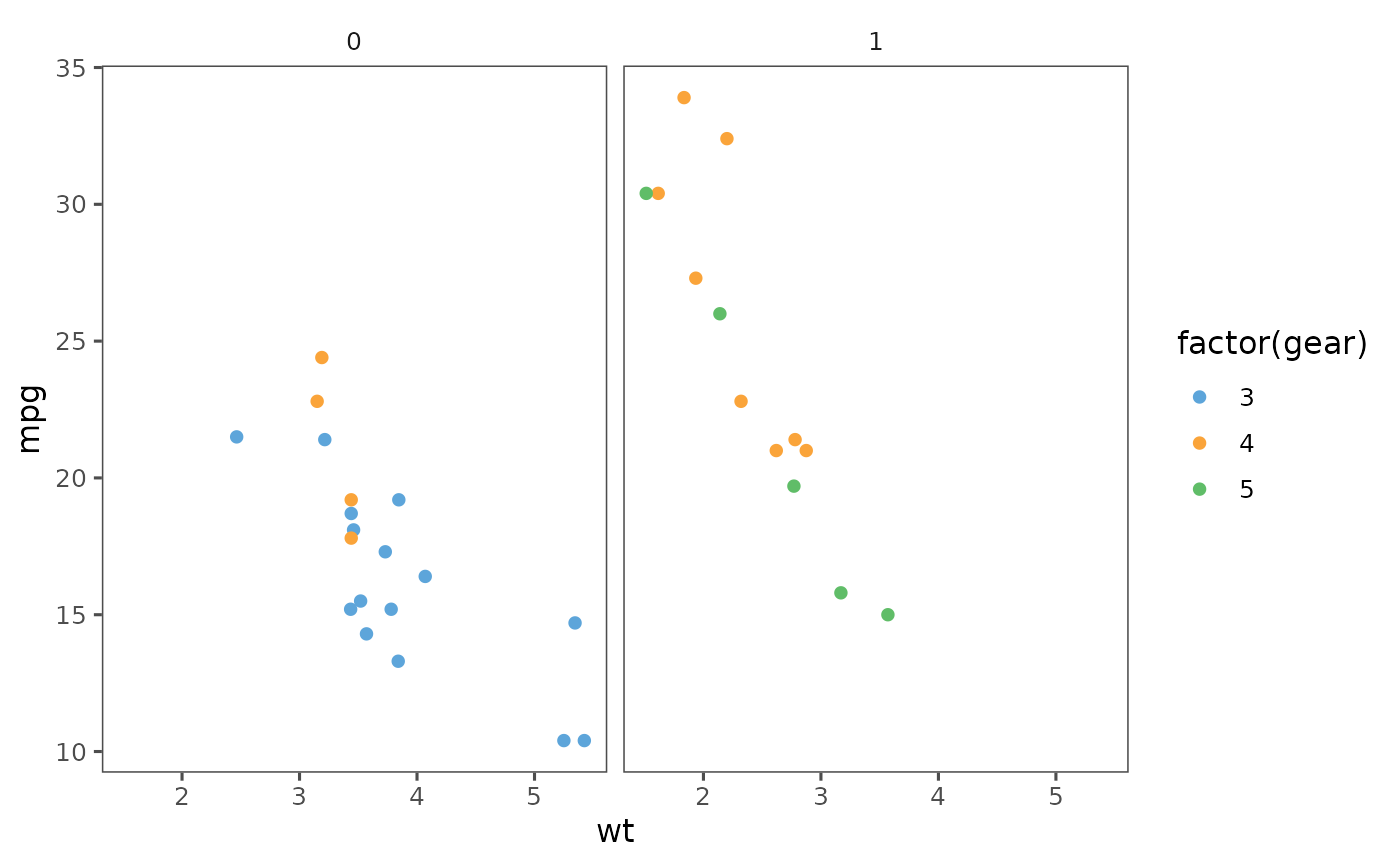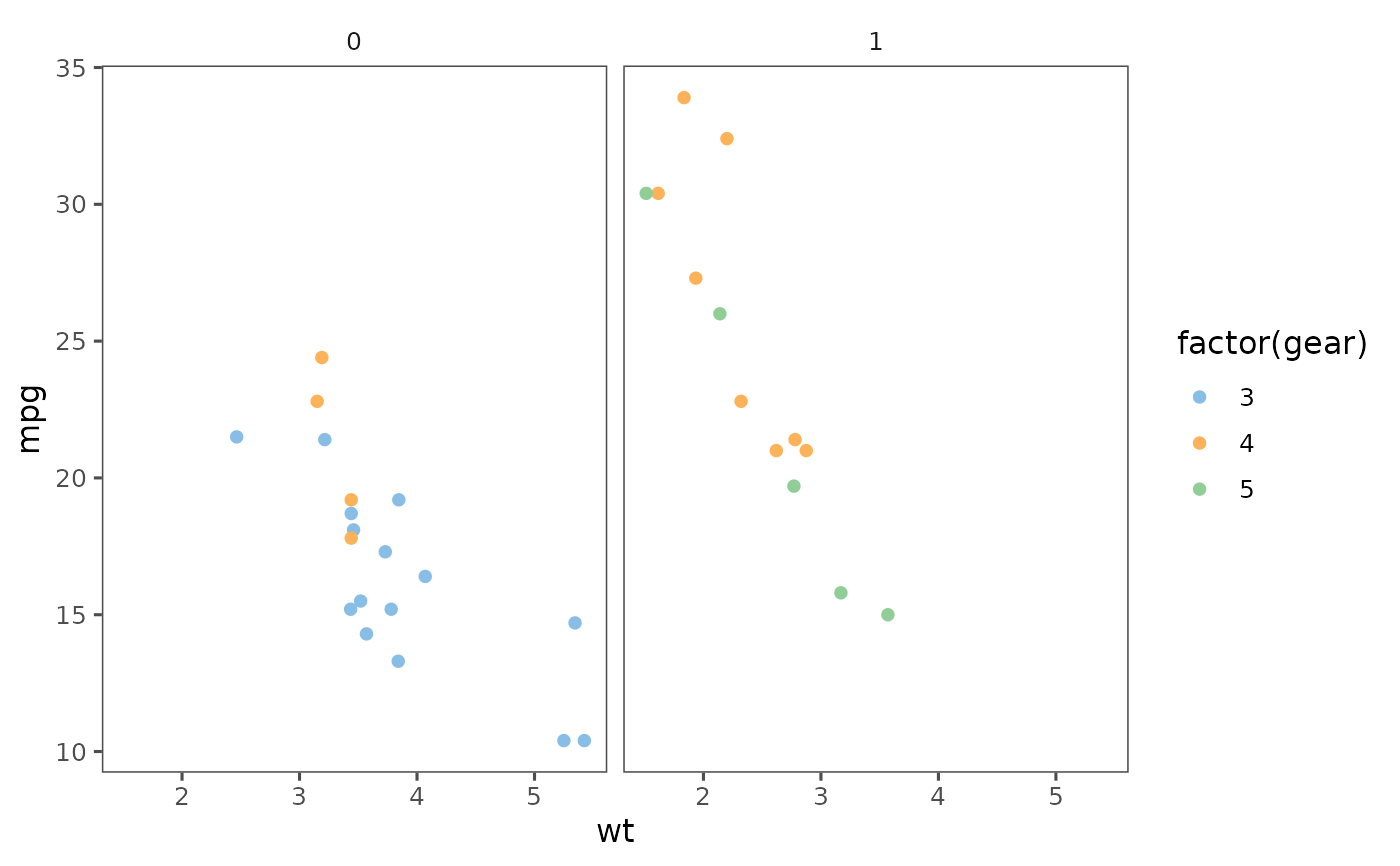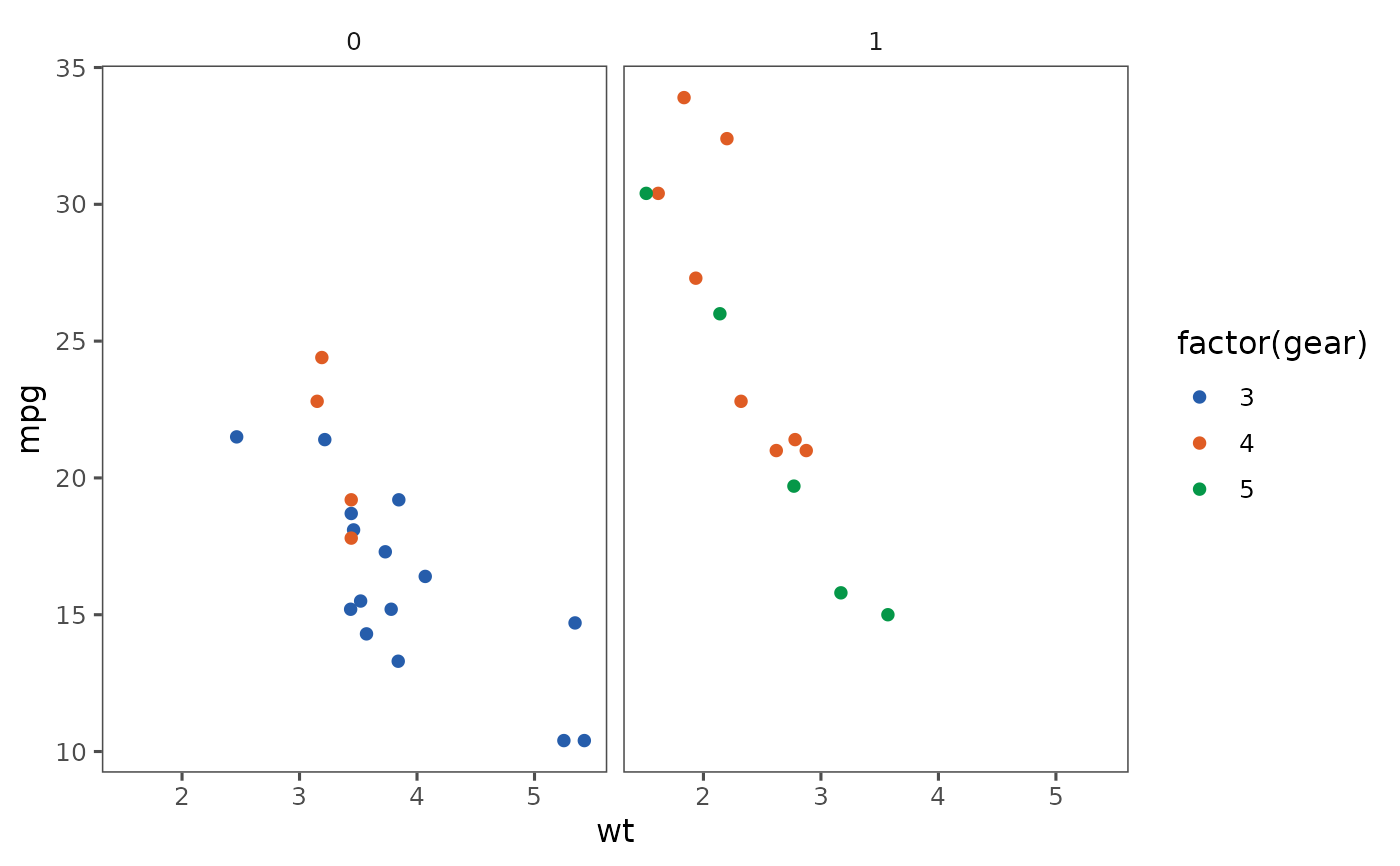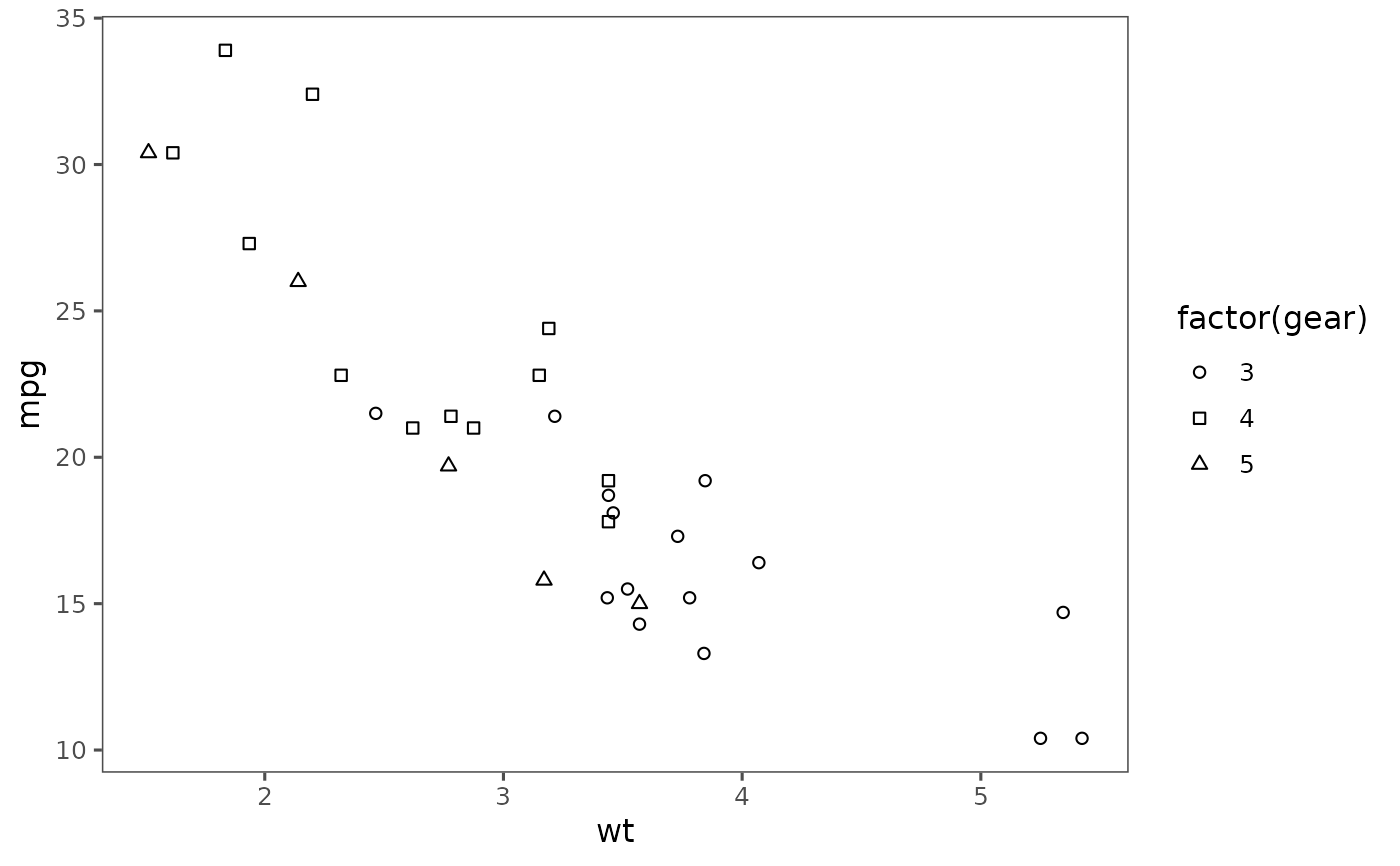Theme based on the rules and examples from Stephen Few's Show Me the Numbers and "Practical Rules for Using Color in Charts".

theme_few(base_size = 12, base_family = "")

## Arguments

base_size base font size, given in pts. base font family

Few, S. (2012) Show Me the Numbers: Designing Tables and Graphs to Enlighten. 2nd edition. Analytics Press.

Stephen Few, "Practical Rules for Using Color in Charts", https://www.perceptualedge.com/articles/visual_business_intelligence/rules_for_using_color.pdf.

## Examples

library("ggplot2")

p <- ggplot(mtcars) +
geom_point(aes(x = wt, y = mpg, colour = factor(gear))) +
facet_wrap(~am)
p + theme_few() + scale_colour_few()p + theme_few() + scale_colour_few("Light")p + theme_few() + scale_colour_few("Dark")ggplot(mtcars) +
geom_point(aes(x = wt, y = mpg, shape = factor(gear))) +
theme_few() +
scale_shape_few()